# The Elements Of Geometry Solution of TS & AP Board Class 9 Mathematics

###### Exercise 3.1

Question 1.

i. How many dimensions a solid has?

ii. How many books are there in Euclid’s Elements?

iii. Write the number of faces of a cube and cuboid.

iv. What is sum of interior angles of a triangle?

v. Write three un-defined terms of geometry.

i. A solid has 3 dimensions, namely length, breadth and height.

ii. Euclid of Alexandria in Egypt wrote 13 books called ‘The Elements’.

iii. A cube is also a cuboid having 6 faces. Hence, both cube and cuboid have 6 faces.

iv. By equivalent of fifth postulate, we know that the sum of angle of a triangle is a constant and is equal to two right angles.

∴ Sum of interior angles of a triangle = 180°

v. In geometry, a point, a line and a plane are the un-defined terms.

Question 2.

State whether the following statements are true or false? Also give reasons for your answers.

a) Only one line can pass through a given point.

b) All right angles are equal.

c) Circles with same radii are equal.

d) A finite line can be extended on its both sides endlessly to get a straight line.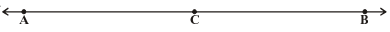e) From the figure, AB > AC

a) False

It is false because through a given point, infinite number of lines can pass.

b) True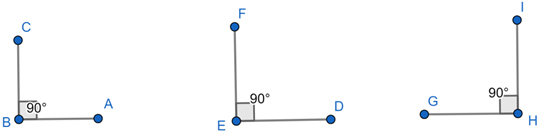Let us consider ∠ABC = 90°, ∠DEF = 90° and ∠GHI = 90°.

Here, ∠ABC = ∠DEF = ∠GHI

i.e. all the right angles are equal (By Postulate 4).

c) True

Two circles coincide if we superimpose the region bounded by one circle on the other circle. Then, their centers and boundaries also coincide.

∴ Circle with same radii will be equal.

d) True

It is true by Euclid’s postulate 2.

e) True

C is the midpoint of line segment AB dividing the lines into two halves i.e. AC = BC

⇒ AB = AC + BC

∴ AB > AC

Question 3.

In the figure given below, show that length AH > AB + BC + CD.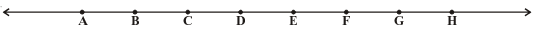Given figure is drawn above.

By Euclid’s Axiom 5,

We know that ‘The whole is greater than the part’.

In the figure, AH is whole.

AB, BC, CD are parts.

⇒ AB + BC + CD is also a part.

∴ AH > AB + BC + CD

Question 4.

If a point Q lies between two points P and R such that PQ = QR, prove that PQ = 1/2 PR.

Given, a point Q lies between 2 points P and R such that PQ = QR.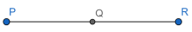⇒ PQ + PQ = QR + PQ

⇒ 2PQ = PR

∴ PQ = 1/2 PR

Hence proved.

Question 5.

Draw an equilateral triangle whose sides are 5.2 cm each.

Step 1:

Draw a line segment AB of length 5.2 cm.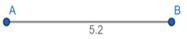Step2:

From Euclid’s third postulate, we know that we can draw a circle with any center and any radius.

Draw a circle with center A and radius AB.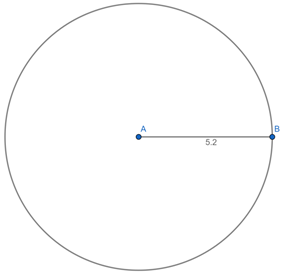Step 3:

Draw another circle with center B and radius BA.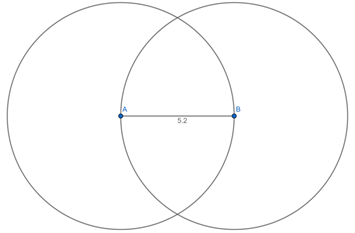Step 4:

The two circles meet at C. Join C to A and B to form ΔABC.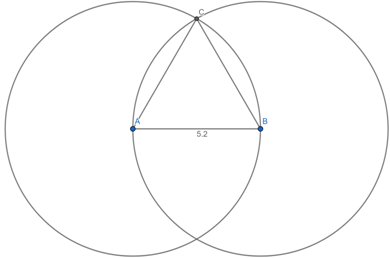Thus, the required equilateral triangle is constructed.

Question 6.

What is a conjecture? Give an example for it.

Sometimes a certain statement that we think is to be true but that is an educated guess based on observations. Such statements which are neither proved nor disproved are called conjectures (hypothesis). Mathematical discoveries often start out as conjectures.

Example: “Every even number greater than 4 can be written as sum of two primes” is a conjecture stated by Gold Bach.

Question 7.

Mark two points P and Q. Draw a line through P and Q. Now how many lines are parallel to PQ, can you draw?

Let us mark 2 points P and Q. Then, draw a line through P and Q.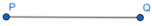There can be infinite number of parallel lines to PQ. So, we cannot draw all of them.

Question 8.

In the adjacent figure, a line n falls on lines l and m such that the sum of the interior angles 1 and 2 is less than 180°, then what can you say about lines l and m.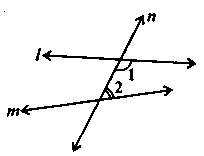By Euclid’s Postulate 5,

We know that if a straight line falling on two straight lines makes the interior angles on the same side of it taken together less than two right angles, then the two straight lines, if produced indefinitely, meet on that side on which the sum of angles is less than two right angles.

Given, a line n falls on lines l and m such that the sum of the interior angles 1 and 2 < 180° on the right side of line n.

So, the lines l and m will eventually intersect on the right side of line n.

Question 9.

In the adjacent figure, if ∠1 = ∠3, ∠2 = ∠4 and ∠3 = ∠4, write the relation between∠1 and ∠2 using a Euclid’s postulate.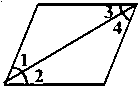Given,

∠1 = ∠3, ∠3 = ∠4 … (i)

And ∠2 = ∠4 … (ii)

By Euclid’s axiom 1,

We know that things which are equal to the same things are equal to one another.

∴ From equation (i), ∠1 = ∠4 … (iii)

Then, from equations (ii) and (iii) and by axiom 1,

⇒ ∠1 = ∠2

Question 10.

In the adjacent figure, we have BX = 1/2 AB, BY = 1/2 BC and AB = BC. Show that BX = BY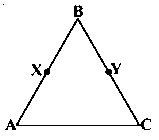Given, AB = BC … (i)

And BX = 1/2 AB and BY = 1/2 BC … (ii)

By Euclid’s axiom 7,

We know that things which are halves of the same thing are equal to one another.

From (i) and (ii),

∴ BX = BY

## MATHS VIDEOS